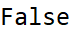# Wolfram CloudResource Object

Function Resource

# GaussianIntegerQ

Determines if a number is a gaussian integer, or not

 ResourceFunction["GaussianIntegerQ"][z] gives True is z is a gaussian integer and False otherwise.

## Details and Options

A gaussian integer is a number whose real and imaginary parts are both integers.

## Examples

### Basic Examples

Zero is a gaussian integer:

 In:=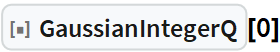Out=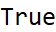Any integer is a gaussian integer:

 In:=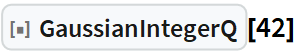Out=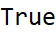A complex number with integer real and imaginary parts is a gaussian integer:

 In:=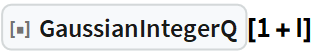Out=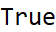Non-integer numbers are not gaussian integers:

 In:=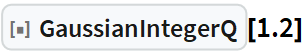Out=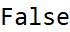In:=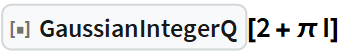Out=